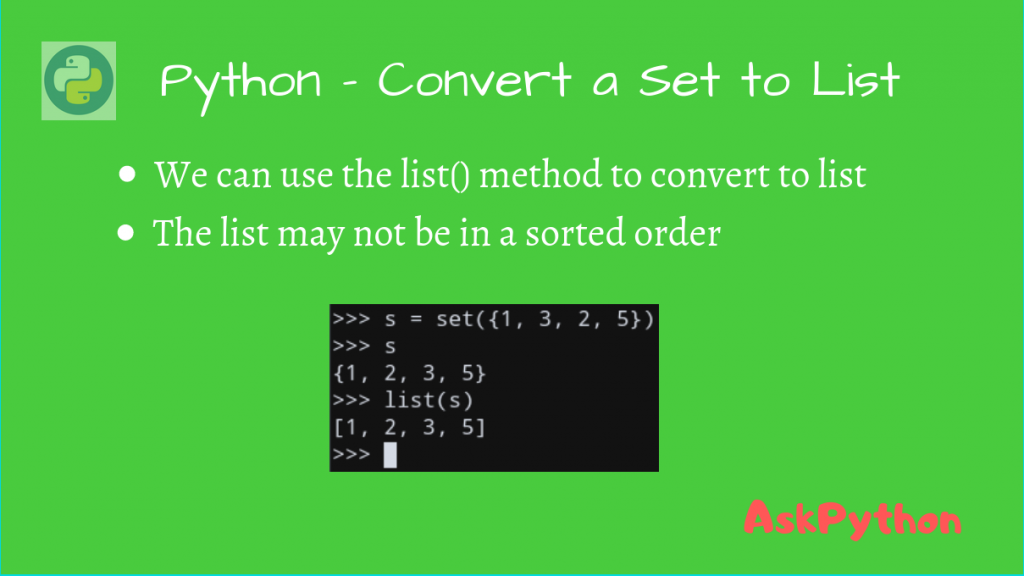# How to Convert a Set to List in Python?We can convert a Set to List in Python using the built-in list() method. Let’s take a look at some examples using this function.

Python offers a range of versatile data structures, each with its own unique features and capabilities. Among these, sets and lists hold a key place due to their wide usage in a variety of applications.

Converting between these two data types is a common task for Python programmers. If you’re looking to gain mastery over such conversions, you’re in the right place. This guide will walk you through various methods to convert a set to a list in Python, offering detailed explanations and examples to make the process clear and straightforward

Python helps you convert a set to a list using built-in methods like list() or through manual iteration. Even frozensets, an immutable variant of sets, can be converted into lists. Let’s delve into these conversion methods and understand their workings

## 2 Methods for Converting Sets to Lists in Python

Python offers various ways to convert a set to a list, ranging from direct in-built methods to explicit looping constructs. This section will guide you through some of these methods and explain how to use them effectively. Each of these methods can be beneficial in different scenarios, depending on the specific requirements of your code.

### 1. Using list() Function

Python list() function takes an iterable as an argument and converts that into a List type object. This is a built-in method ready for you to use. As sets in Python are iterable, we can utilize this function for our set-to-list conversion. Let’s look at how we can use this to convert set to a list.

```my_list = list(my_iterable)
```

Since a set is also iterable, we can pass it into the `list()` method and get our corresponding list.

```# Create a Python set
my_set = set({1, 4, 3, 5})
# Convert the set into a list
my_list = list(my_set)

print(my_list)
```

The output, as expected, is a list containing the above values.

```[1, 3, 4, 5]
```

Note that the order of the list can be random, and not necessarily sorted.

For example, take the below snippet.

```s = set()

print(list(s))
```

Output in my case:

```['B', 'A']
```

### 2. Using Manual Iteration

Another method of converting a set to a list is by manually iterating through the set and adding elements to a list. Although this method is more verbose and doesn’t provide any real-world advantage over the list() method, it provides a clear illustration of how iteration works in Python.

It can be useful in situations where more complex operations need to be performed on each element during the conversion process.

```s = set({1, 2, 3})

a = []

for i in s:
a.append(i)

print(a)
```

Again, the output is a list:

```[1, 2, 3]
```

## Convert a frozenset to a list

A frozenset is a built-in immutable set type, meaning its elements cannot be modified after creation. However, just like regular sets, frozensets can also be converted into lists. The conversion process is identical to that of a regular set, providing a uniform method of converting both mutable and immutable set types into a list. This extends the flexibility and power of Python’s data-handling capabilities.

```f_set = frozenset({1, 3, 2, 5})

a = list(f_set)

print(a)
```

Output

```[1, 2, 3, 5]
```

## Conclusion

Understanding how to convert a set into a list, a fundamental aspect of Python programming, is an essential skill for any developer. The methods discussed in this tutorial will certainly come in handy while dealing with such data structures in Python. What other Python data conversions do you think would be helpful to learn about?